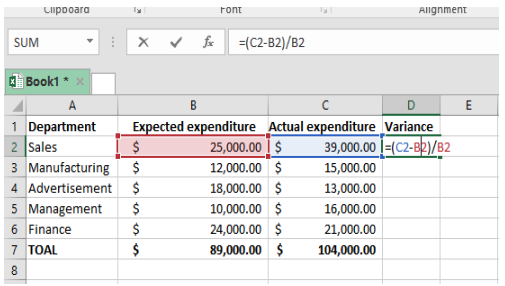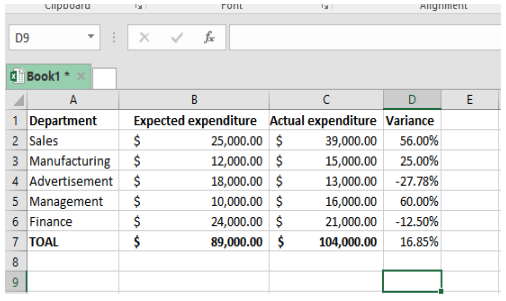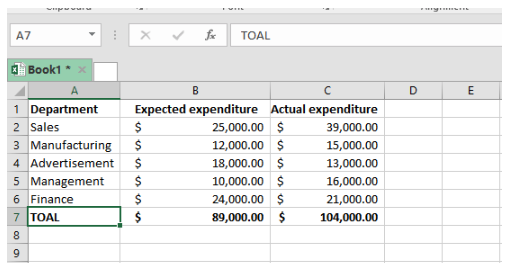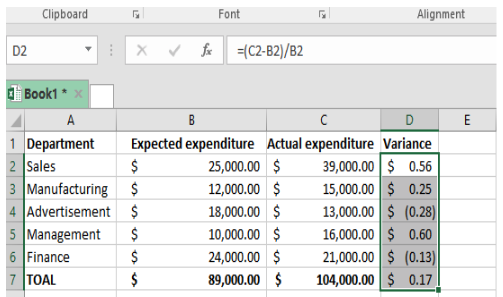Get instant live expert help with Excel or Google Sheets“My Excelchat expert helped me in less than 20 minutes, saving me what would have been 5 hours of work!”

#### Post your problem and you'll get expert help in seconds

Your message must be at least 40 characters
Our professional experts are available now. Your privacy is guaranteed.

# How to Calculate the Percent Variance in Excel

A percent variance is a proportional change in an account between two reporting periods. You can calculate this in Excel with the right formula.

## Calculate the percent variance in Excel

### Formula

The general formula to calculate percent variance in Excel is:

`=(new  - original) / original`

### Explanation of formula

The percent variance formula is used to calculate the difference between the values of any two periods, such as this year and last year, the variance between budgeted values and actual values and many others.

To understand the working of this formula appropriately, we need to take a baseline value and a new value. After getting the difference between the baseline value and the new value, we have to divide the answer with the baseline or original value. To get the result in percentage, we format it using the percent.

### Example 1

Assuming that the original value or baseline value is 400 and the new value is 600, the difference shall be 200. I.e.

=(600-400)

Divide the difference by the original value.

200/400 = 0.5

To get percent variance, we multiply the answer by 100% as shown below

0.5*100=50%

### Example 2

We want to calculate the percent variance between the expected expenditure and the actual expenditure in the worksheet below. In this example, we shall create another column where we shall have the variance. Let it be column D.Figure 1: Calculating variance in Excel

In the cell D2, specify the following formula:

`=(C2-D2)/D2`Figure 2: Getting the difference before calculating the variance

Then, press Enter and drag the formula down across all the other cells. This will give you the variance of each entry.Figure 3: Drag the formula across other cells to get their variance.

Note that the entries in brackets in column D show a negative difference. To get the percent variance, we now need to format the variance column as the percentage. This will give you the results as shown in figure 4 below.Figure 4: Calculate percent variance

Still need some help with Excel formatting or have other questions about Excel? Connect with a live Excel expert here for some 1 on 1 help. Your first session is always free.

### Did this post not answer your question? Get a solution from connecting with the expert.Another blog reader asked this question today on Excelchat:0 Followers

Most reacted comment
2 Comment authorsRecent comment authorsGuest
Julie

What if you have 3 columns you need to get the percentage variance on? D6 = \$269,760, E6= \$255,809, & F6= \$291,840. You are only showing 2 columns being used, I need to know if I just make the formula like this or if there is a different formula. my formula =(F6-E6-D6)/D6????Raghav

Hey Julie, thanks for you question! Myself and other Excel experts who write these blogs are also online and available to help. I can actually help you out on your specific problem on percent variance – just post it in the question box on our adjoining sister site excelchat.co (www.excelchat.co). If I’m not able to jump on to start a session with you, one of my colleagues will in less than a minute after you post. Because you found us through this blog, your first help session is on us. Just sign up with your same email 🙂 Let me… Read more »

## Subscribe to Excelchat.coAnother blog reader asked this question today on Excelchat: